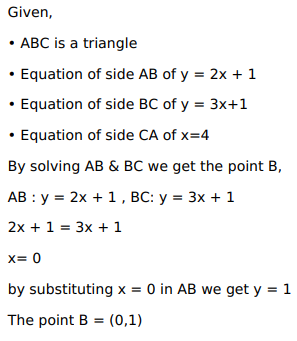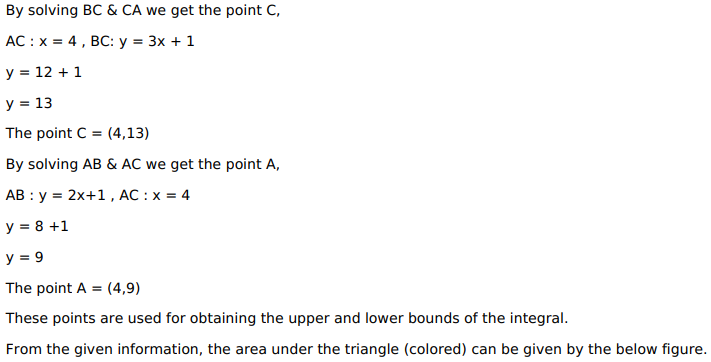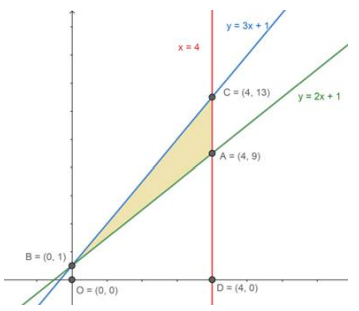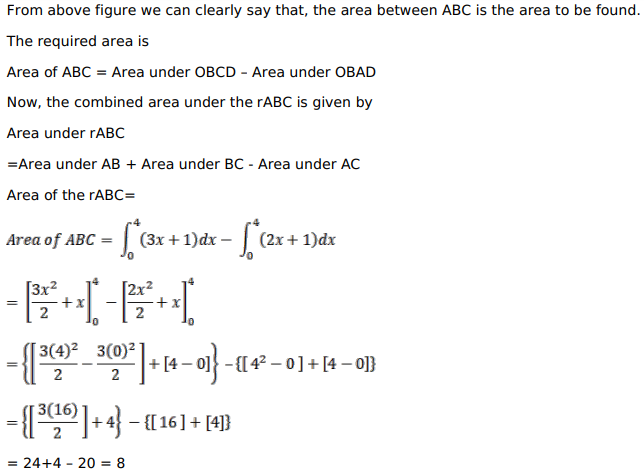# Using integration, find the area of the triangle, the equations of whose sides areQuestion:

Using integration, find the area of the triangle, the equations of whose sides are $y=2 x+1, y=3 x+1$ and $x=4$.

Solution: